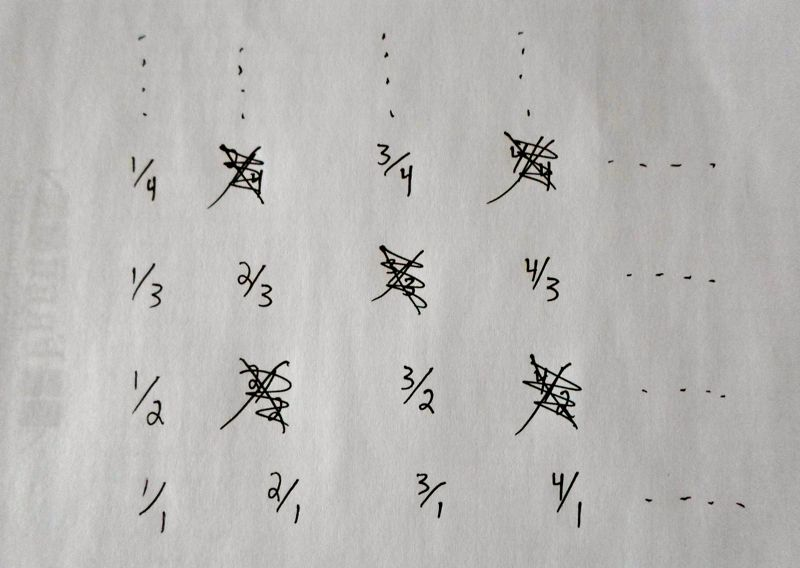# In-Depth

A more in-depth discussion of $$|\mathbb{N}\times\mathbb{N}| = |\mathbb{N}|$$.

As for proving $$|\mathbb{N}\times\mathbb{N}| = |\mathbb{N}|$$, the argument given in the short explanation is rigorous and complete. The one-to-one correspondence between $$\mathbb{N}$$ and $$\mathbb{N}\times\mathbb{N}$$ is given by listing off elements of $$\mathbb{N}\times\mathbb{N}$$ in a systematic way, using their representation as a lattice of points filling the upper-right quadrant of the plane and working out in zigzags from the origin.

Here we'll discuss how the same proof can be altered to calculate the size of the set of all fractions.

Consider the set of all positive fractions: all numbers of the form $$\frac{a}{b}$$, where $$a$$ and $$b$$ are natural numbers, but we don't let $$b=0$$ (this is never allowed; dividing by zero is a no-no) or $$a=0$$ (because we want positive fractions). Let's call the set of all positive fractions $$\mathbb{Q}^+$$.

If we also allow for negative fractions and zero, this set of all fractions (those of the form $$\frac{a}{b}$$, where $$a$$ and $$b$$ are any integers except $$b$$ can't be zero) is called the irrational numbers, and conventionally denoted by $$\mathbb{Q}$$.

Because each natural number can be written as such a fraction, for example $$5 = \frac{5}{1}$$, we can think of the set of natural numbers as a subset of the set of positive fractions. So $$\mathbb{Q}^+$$ is an infinite set, and it is at least as large as $$\mathbb{N}$$.

A natural question to ask is: are $$\mathbb{N}$$ and $$\mathbb{Q}^+$$ the same size? That is, can we construct a one-to-one correspondence between $$\mathbb{N}$$ and $$\mathbb{Q}^+$$?

The answer is yes, and we will show this using a variation of the zigzag trick.

Imagine assigning fractions to the lattice of points in the upper-right quadrant of the plane, as in the following picture.Every positive fraction will appear somewhere in this array -- specifically, the fraction $$\frac{a}{b}$$ is in column $$a$$ and row $$b$$, counting up and right. The only problem is, some fractions appear more than once! This is because, for example, $$\frac{2}{1} = \frac{4}{2}$$, or $$\frac{1}{1} = \frac{2}{2} = \frac{3}{3} = \frac{4}{4}$$, etc.

So we do something ad hoc, and just cross out all the duplicates in this array. This amounts to crossing out all the fractions that aren't in reduced form, i.e. aren't as simplified as possible. The picture might look like this.And now we use the zigzag trick to list out all the positive fractions, but skipping over the crossed-out terms. The first part of this list might look like the following.

$0 \leftrightarrow 1/1\\ 1 \leftrightarrow 2/1\\ 2 \leftrightarrow 1/2\\ 3 \leftrightarrow 1/3\\ 4 \leftrightarrow 3/1\\ 5 \leftrightarrow 4/1\\ 6 \leftrightarrow 3/2\\ 7 \leftrightarrow 2/3\\ 8 \leftrightarrow 1/4$

This list gives us a one-to-one correspondence between $$\mathbb{N}$$ and $$\mathbb{Q}^+$$. Every natural number is put in correspondence with a positive fraction; every positive fraction gets hit once and only once.

Therefore $$|\mathbb{N}| = |\mathbb{Q}^+|$$.

Remember in the Basics section, we showed that the natural numbers $$\mathbb{N}$$, which are non-negative, were the same size as the integers $$\mathbb{Z}$$, which are positive or negative or zero. This involved listing out the positives and negatives in alternation. Now this same trick can be used to extend the proof we just gave, of $$|\mathbb{N}| = |\mathbb{Q}^+|$$, to actually show that $$|\mathbb{N}| = |\mathbb{Q}|$$.

That is, the natural numbers are the same size as the set of all fractions, the irrational numbers.

In the third module, we'll say more about the irrationals and how they defy our intuition.

Top